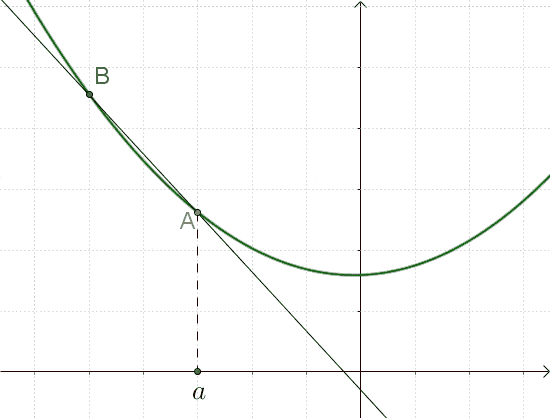# An intuitive understanding for derivatives

• B
• sahilmm15
In summary, the conversation discusses the concept of derivatives and how they are represented by the equation ##\frac{dy}{dx} = 5## in the given linear equation ##y=5x+3##. The conversation also touches on the importance of understanding mathematics intuitively and paying homage to great mathematicians such as Weierstraß and Hardy.f

#### sahilmm15

Given, $$y=5x+3$$. We need to find how ##y## would change when we would make a very small change in ##x##.
So, if we assume the change in ##x## to be ##dx## the corresponding change in ##y## would be ##dy##.So, $$y+dy = 5(x+dx)+3$$ From here we get
$$\frac{dy}{dx}=5$$ From mathematical point of view everything is clear, but can you make me understand this $$\frac{dy}{dx}=5$$ in a more intuitive
way(graphical maybe) or something else.

This isn't the best example to show what's going on, since the derivative is constant and the tangent coincides with the curve. The slope (5) of the straight does not change when you approach a certain point. A more intuitive example might be this picture: (https://fr.wikipedia.org/wiki/Tangente_(géométrie))•sahilmm15
I'd say you are at the inituitive bedrock there. ##y = 5x + 3## describes a linear relationship where the change in ##y## is ##5## times the change in ##x##.

•sahilmm15
I'd say you are at the inituitive bedrock there. ##y = 5x + 3## describes a linear relationship where the change in ##y## is ##5## times the change in ##x##.
##dy=5dx## does it mean if there is a very small change of x say, 'a' units then the corresponding change in y would be 5 times of that'a' unit.

##dy=5dx## does it mean if there is a very small change of x say, 'a' units then the corresponding change in y would be 5 times of that'a' unit.
Intuitively, yes. Note that a linear relationship (straight line) is simple. It's really when you look at the tangent to a curve that something mathematically sophisticated is needed.

•sahilmm15
Intuitively, yes. Note that a linear relationship (straight line) is simple. It's really when you look at the tangent to a curve that something mathematically sophisticated is needed.
Thanks for the help, intuitively understanding math is fun. Now, this would always give me a sense of what's really going on while doing problems.

##dy=5dx## does it mean if there is a very small change of x say, 'a' units then the corresponding change in y would be 5 times of that'a' unit.
I like Weierstraß' way of writing it: ##\mathbf{y(x_{0}+v)=y(x_{0})+J(v)+r(v)}##

##\dfrac{dy}{dx}\cdot dx=dy=5\cdot(x_0+dx)+3=(5x_0+3)+\mathbf{J}(dx)+0 \Longrightarrow \mathbf{J}(dx)=5\cdot dx## and the derivative / gradient / Jacobi matrix turns out to be the linear function 'times five'.

I like Weierstraß' way of writing it: ##\mathbf{y(x_{0}+v)=y(x_{0})+J(v)+r(v)}##

##\dfrac{dy}{dx}\cdot dx=dy=5\cdot(x_0+dx)+3=(5x_0+3)+\mathbf{J}(dx)+0 \Longrightarrow \mathbf{J}(dx)=5\cdot dx## and the derivative / gradient / Jacobi matrix turns out to be the linear function 'times five'.
I wonder if there is a town in Germany anywhere that, as a homage to the great mathematician, has a Weierstraßstraße?

I wonder if there is a town in Germany anywhere that, as a homage to the great mathematician, has a Weierstraßstraße?
There is a Weierstaßweg in his birth town Ostenfelde, better village in the middle of nowhere, since it has only 2,500 inhabitants, and several others in the near neighborhood. He is a bit underestimated here I think, because best mathematicians can hope for to be remembered by non mathematicians is Gauß. I wonder if people - not mathematicians of course - remembered Hardy?

•PeroK
There is a Weierstaßweg in his birth town Ostenfelde, better village in the middle of nowhere, since it has only 2,500 inhabitants, and several others in the near neighborhood. He is a bit underestimated here I think, because best mathematicians can hope for to be remembered by non mathematicians is Gauß. I wonder if people - not mathematicians of course - remembered Hardy?
People in the UK remember Laurel & Hardy, but not Littlewood & Hardy!

•fresh_42